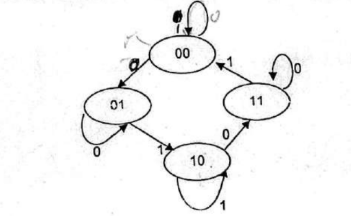### Exam Year

Tribhuvan University

Institute of Science and Technology

2075

Bachelor Level / first-semester / Science

Computer Science and Information Technology( CSC111 )

Digital Logic

Full Marks: 60 + 20 + 20

Pass Marks: 24 + 8 + 8

Time: 3 Hours

Candidates are required to give their answers in their own words as far as practicable.

The figures in the margin indicate full marks.

Group A

Attempts any two Questions (2 x 10 = 20)

1

Implement the following function F = Σ(1, 2, 3, 4, 8) using

1. Decoder
2. Multiplexer
3. PLA
2

Design clocked sequential circuit of the following state diagram by using JK flip-flop3

The following is a truth table of a 3-input,4 output combinational circuit. Tabulate the PAL programming table for the circuit and mark for the circuit and mark the fuses to be blown in a PAL diagram.

 Input Output X Y Z A B C D 0 0 0 0 0 0 0 0 0 1 1 1 1 1 0 1 0 1 0 1 1 0 1 1 0 1 0 1 1 0 0 1 0 1 0 1 0 1 0 0 0 1 1 1 0 1 1 1 0 1 1 1 0 1 1 1

Group B

Attempts any eight question (8 x 5 = 40)

4

Convert the following decimal numbers to the indicated bases.

1. 7562.45 to octal
3. 175.175 to binary
5

Express the Boolean Function F = A + B’ C in a sum of min terms .

6

Reduce the following function using k-map F = B’D + A’BC’ + AB’C + ABC’

7

Design a combinational circuit with three inputs, x, y, and z, and three outputs, A, B, and C. When the binary input is 0, 1, 2, or 3, the binary output is one greater than the input. When the binary input is 4, 5, 6, or 7, the binary output is one less than the input.

8

Implement half adder using 2-4 decoder.

9

What is the difference between a serial and parallel transfer? Explain how to convert serial data to parallel and parallel data to serial. What type of register is needed?

10

Design 4-bit even parity generator.

11

Design a 4-bit binary ripple counter with D flip-flops.

12

Write short notes on any two:

1. SIMM
2. RTL
3. Parity Checker